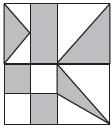# User Forum

Subject :NSO    Class : Class 7

What fraction of the given figure is shaded ?(A) 7/16

(B) 1/2

(C) 9/16

(D) 17/32
pls explain how it is 1/2

## Ans 1:

Class : Class 8
Divide each square into four parts just like the one of them in the figure. Total 16 squares will be there. Now int the first box there two whole squares and two halves forming (the triangle) one more square. In the second box there is one whole square and two halves forming one more square. Next in the third box there are already two box shaded. In the last box if the triangle is divided into pieces and formed into a square one more square is found. So total there are 8 squares shaded out of 16 squares which is 8/16 which when divided further is 1/2.

Class : Class 8

Class : Class 7
good